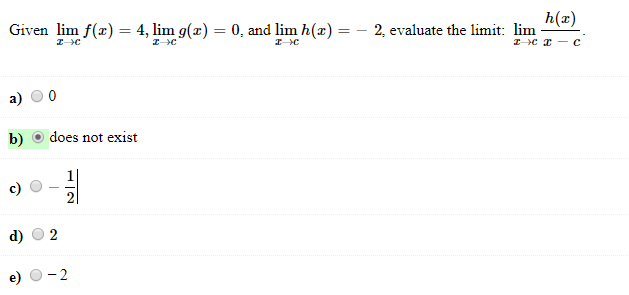Given lim f(z) = 4, lim g(z) = 0, and lim h (z) =-2, evaluate the limit:h(x)lima) 0b) does not existc)d) O2e) O-2

Question

Solvehelp_outlineImage TranscriptioncloseGiven lim f(z) = 4, lim g(z) = 0, and lim h (z) =-2, evaluate the limit: h(x) lim a) 0 b) does not exist c) d) O2 e) O-2 fullscreen
Step 1

Here, objective is to evaluate the limit,

Step 2

from given data, we have

Step 3

consider the ...

Want to see the full answer?

See Solution

Want to see this answer and more?

Our solutions are written by experts, many with advanced degrees, and available 24/7

See Solution
Tagged in

Limits